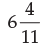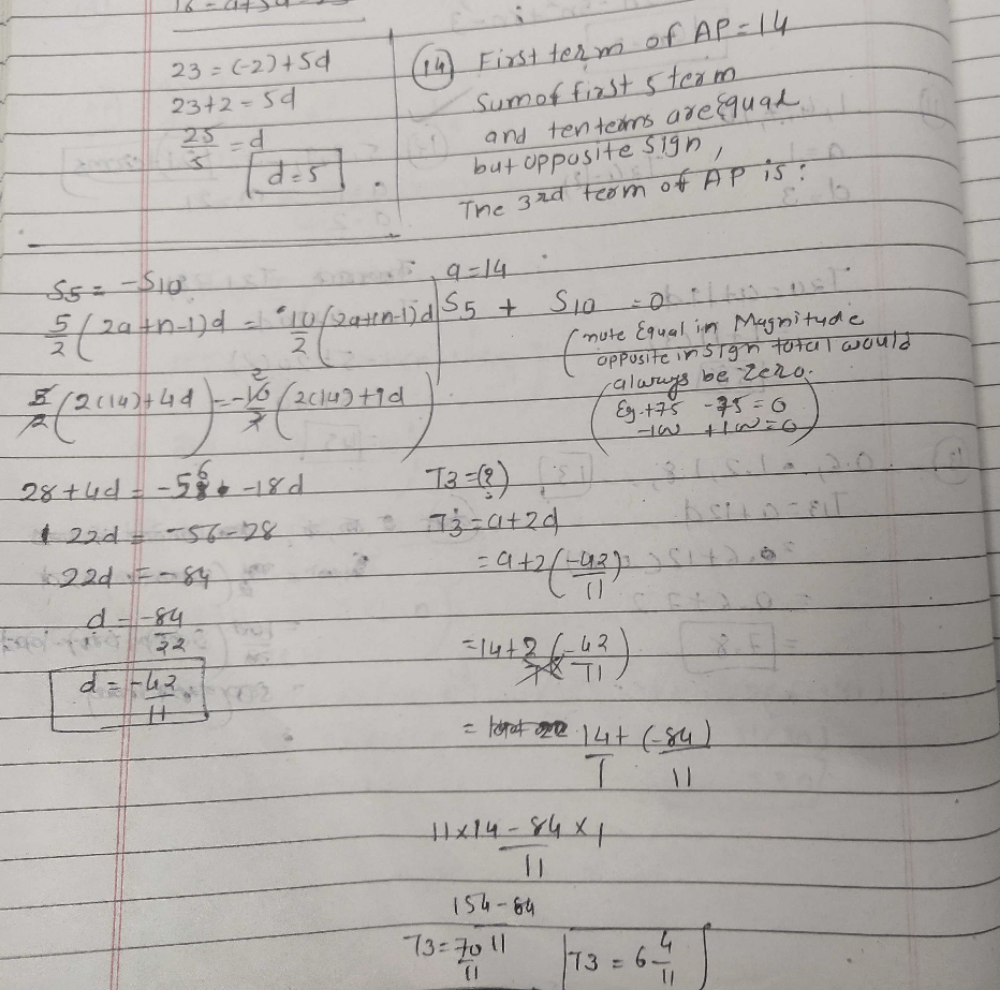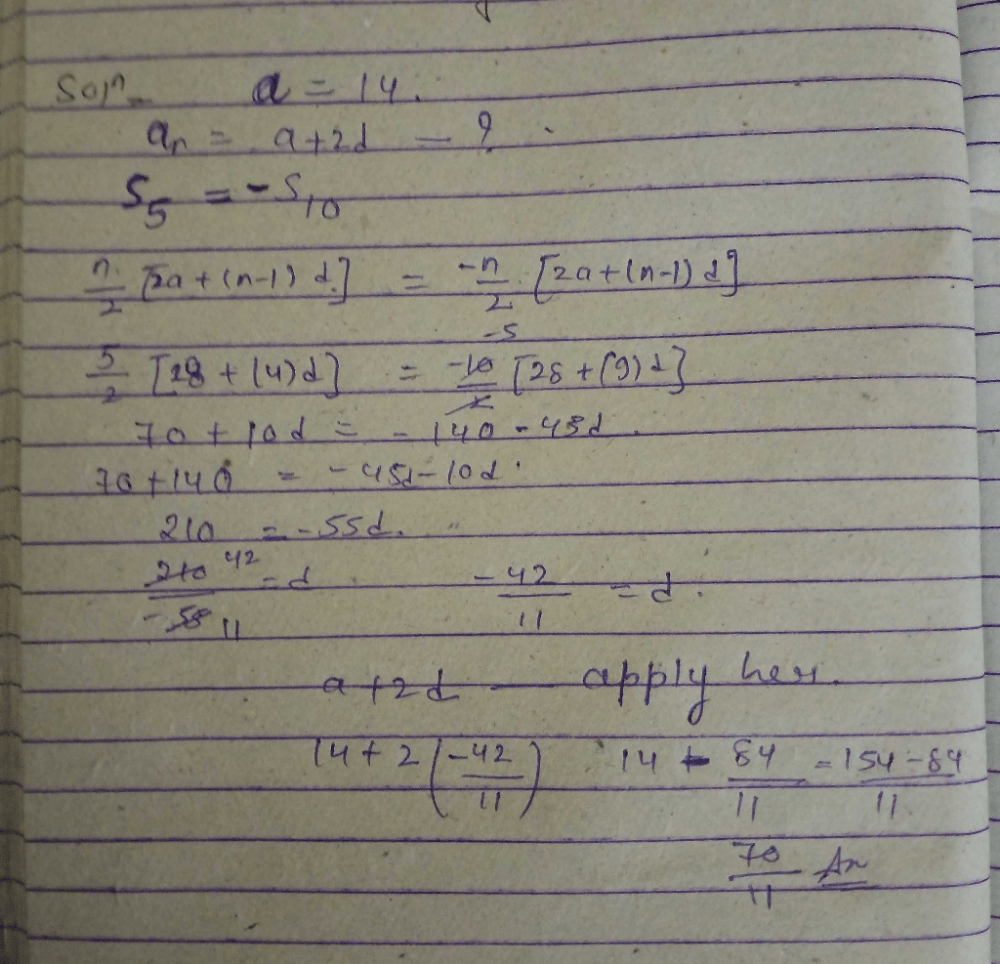Courses

# The first term of an A.P is 14 and the sums of the first five terms and the first ten terms are equal is magnitude but opposite in sign. The 3rd term of the AP isa)b)6c)4/11d)none of theseCorrect answer is option 'A'. Can you explain this answer? Related Test: Test: Arithmetic And Geometric Progressions - 1

## CA Foundation QuestionSagarika Shinde Jul 02, 2018
a₁ = 14
a₅ = a₁ + 4d = 14 + 4d
∑₅ = 5(a₁ + a₅)/2
= 5(28+4d)/2
= 70 + 10d

a₁₀ = a₁ + 9d
∑₁₀ = 10(a₁ + a₁₀)/2
= 10(28+9d)/2
= 140 + 45d

∑₁₀ = -∑₅
140 + 45d = -70 - 10d
55d = -210
d = -210/55 = -42/11

a₃ = a₁ + 2d = 70/11Meet Trivedi Oct 21, 2019First term =14common diff=d==> 5/2(2*14+(4)d)=-10/2(2*4+9d)solve it d=-42/11t3=a+ 2d=70/11Shikha Singh Sep 14, 2020Krishna Singi Nov 08, 2019
Option a